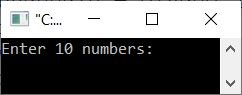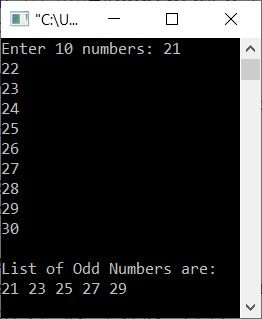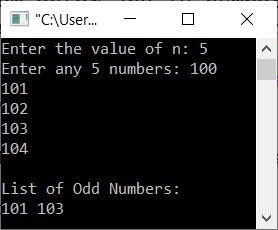# C++ Program to Find and Print Odd Numbers in an Array

This article provides some programs in C++ that find and print all odd numbers in an array entered by the user. The list of programs covered here is as follows:

## Print odd numbers in an array of 10 elements

The question is, "Write a C++ program that receives 10 elements (numbers) from the user at run-time and prints all odd numbers." The program given below is its answer:

```#include<iostream>

using namespace std;
int main()
{
int arr, i;
cout<<"Enter 10 numbers: ";
for(i=0; i<10; i++)
cin>>arr[i];
cout<<"\nList of Odd Numbers are:\n";
for(i=0; i<10; i++)
{
if(arr[i]%2!=0)
cout<<arr[i]<<" ";
}
cout<<endl;
return 0;
}```

The snapshot given below shows the initial output produced by the above C++ program on printing all the odd numbers available in a given array:Enter any 10 numbers, such as 21, 22, 23, 24, 25, 26, 27, 28, 29, and 30, and press `ENTER` key to find and print all odd numbers from these ten numbers, as shown in the screenshot below:## Print odd numbers in an array of n elements

This program lets the user enter both the size (n) and the array elements.

```#include<iostream>

using namespace std;
int main()
{
int n, i;
cout<<"Enter the value of n: ";
cin>>n;
int arr[n];
cout<<"Enter any "<<n<<" numbers: ";
for(i=0; i<n; i++)
cin>>arr[i];
cout<<"\nList of Odd Numbers:\n";
for(i=0; i<n; i++)
{
if(arr[i]%2!=0)
cout<<arr[i]<<" ";
}
cout<<endl;
return 0;
}```

Here is its sample run with user input of 5 as size and 100, 101, 102, 103, and 104 as the five elements or numbers for the array:## Find and Print Odd Numbers Using Another Array

This program uses two arrays: one stores the original array, and the second stores only odd numbers from the original array.

```#include<iostream>

using namespace std;
int main()
{
int n, i, j=0;
cout<<"Enter the value of n: ";
cin>>n;
int arr[n], odd[n];
cout<<"Enter any "<<n<<" numbers: ";
for(i=0; i<n; i++)
{
cin>>arr[i];
if(arr[i]%2!=0)
{
odd[j] = arr[i];
j++;
}
}
if(j==0)
else if(j==1)
cout<<"\nOnly one odd number found. That is:\n"<<odd;
else
{
cout<<"\nList of Odd numbers:\n";
for(i=0; i<j; i++)
cout<<odd[i]<<" ";
}
cout<<endl;
return 0;
}```

Here is its sample run with user input of 4 as size and 100, 102, 103, 104 as four elements or numbers:C++ Quiz

« Previous Program Next Program »